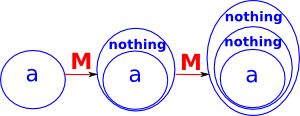# Maths - Category Theory - Identity & Maybe Monads

When we are using a monad in a Haskell program we can take some function of a type 'a':

f :: a -> a

and add some 'effect' to that function:

a -> m a

This effect could be somthing like input/output or state which would otherwise make the program non-pure functional.

On this page we will create instances of the built in Monad class, which is defined in the prelude like this:

 ```class Monad m where return :: a -> m a (>>=) :: m a -> (a -> m b) -> m b```

In the identity monad the modad does not have any additional effect and the function 'f' is applied directly to x:

 ```module Main where instance Monad Ident where return x = x x >>= f = f x ```

 We can introduce a functor which takes any type and adds a 'nothing' value.We can draw this as an endo-functor.#### Maybe - Natural Transformations

 The unit transform adds the 'nothing' value to type 'a'The mult transform combines the inner and outer 'nothing' into a single nothing.```module Main where instance Monad Maybe where return x = Just x (Just x) >>= f = f x Nothing >>= _ = Nothing (Just _ ) >> f = f Nothing >> _ = Nothing Fail _ = Nothing maybe :: b -> (a -> b) -> Maybe a -> b maybe n _ Nothing = n maybe _ f (Just x) = f x```

http://www.mathreference.com/

Where I can, I have put links to Amazon for books that are relevant to the subject, click on the appropriate country flag to get more details of the book or to buy it from them.The Princeton Companion to Mathematics - This is a big book that attempts to give a wide overview of the whole of mathematics, inevitably there are many things missing, but it gives a good insight into the history, concepts, branches, theorems and wider perspective of mathematics. It is well written and, if you are interested in maths, this is the type of book where you can open a page at random and find something interesting to read. To some extent it can be used as a reference book, although it doesn't have tables of formula for trig functions and so on, but where it is most useful is when you want to read about various topics to find out which topics are interesting and relevant to you.